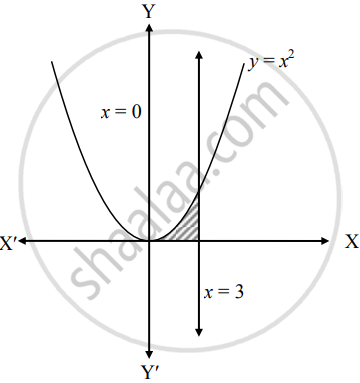# Find the area of the region bounded by the curve y = x2, the X−axis and the given lines x = 0, x = 3 - Mathematics and Statistics

Sum

Find the area of the region bounded by the curve y = x2, the X−axis and the given lines x = 0, x = 3

#### Solution

Let A be the required area.

Consider the equation y = x∴ A = int_0^3 y  "d"x

= int_0^3 x^2  "d"x

= [x^3/3]_0^3

= 1/3(3^3 - 0)

= 9 sq.units

Concept: Area Bounded by the Curve, Axis and Line
Is there an error in this question or solution?